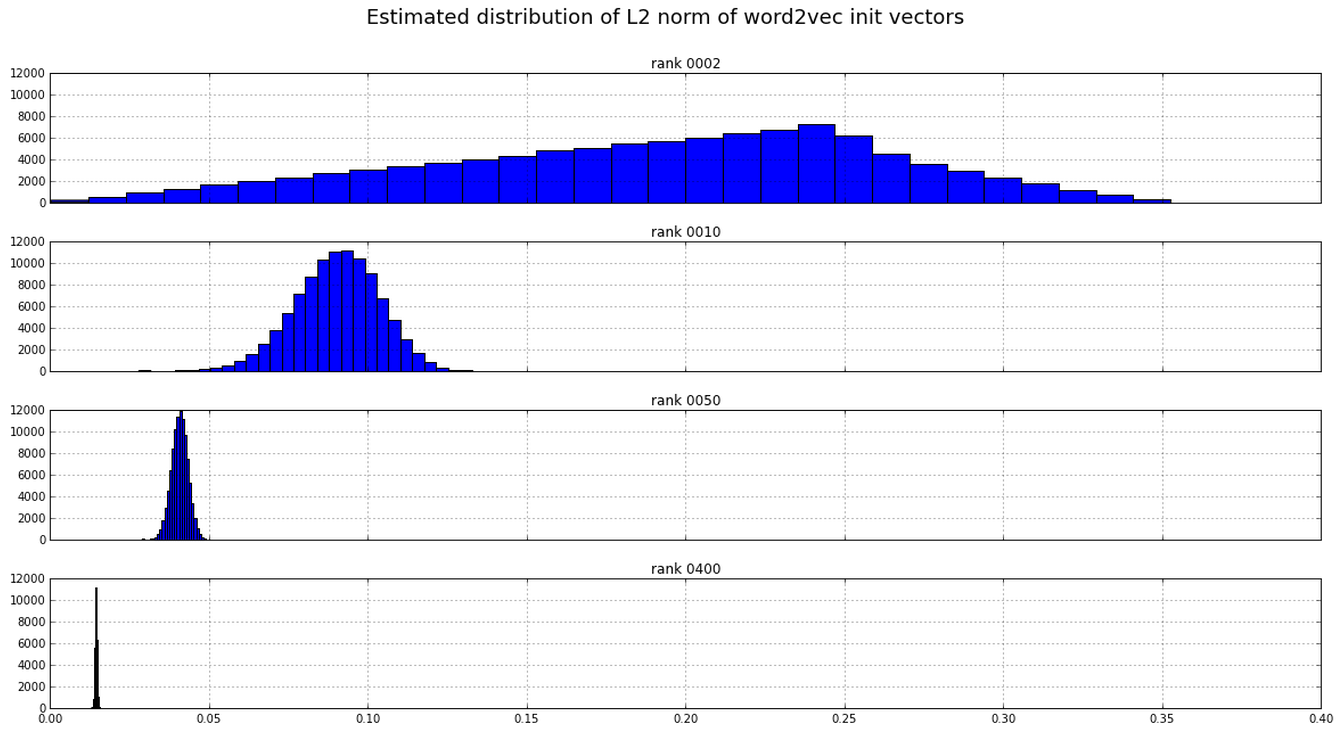# Word2vec weight initialisation

The initialisation of the weights in word2vec is not what I expected.

• syn1 The weights connecting the hidden- to the output-layer are initialised to zero (in both the hierarchical softmax and the negative sampling cases)
• syn0 The initial values for the weights connecting the input- to the hidden-layer are drawn uniformly and independently from the interval $[\frac{-1}{2n}, \frac{1}{2n} ]$, where $n$ is the rank of the hidden layer (i.e. number of hidden units.)

The range of interval from which the syn0 weights are sampled was chosen depends on the rank. I had presumed that this was to account for the dependency of the distribution of the dot product (and in particular the L2-norm) on the rank. However, estimating these distributions empirically, this doesn’t seem to be the case:According to Mikolov (in a helpful response in the word2vec google group), the initialisation of the weights was chosen empirically, since it seemed to work well.

Questions:

1. I was unable to derive an expression for the distribution of L2-norms mathematically. Can someone help with that?